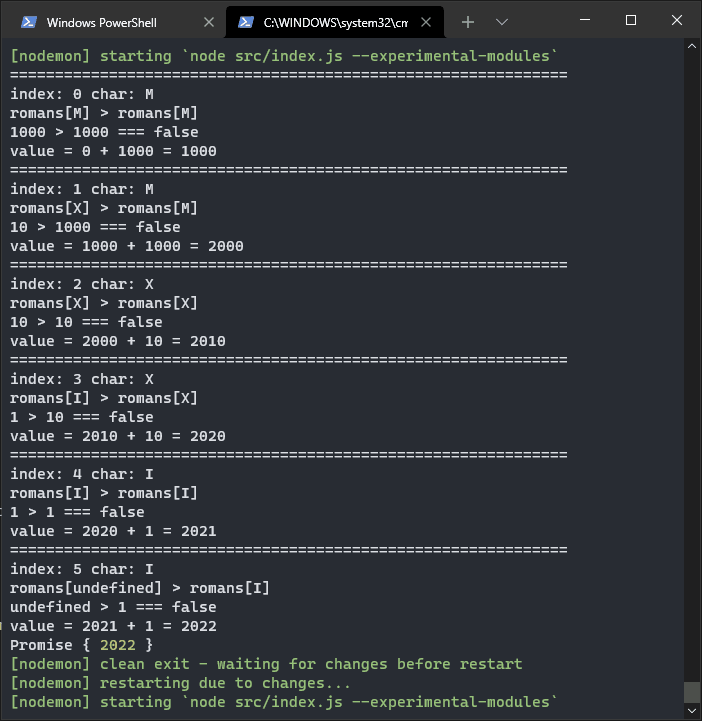The elves are excited about new technologies. They convinced Santa to modernize everything. They are converting millennia of paperwork into digital documents. They realized, however, that the more they go back in time, the more difficult it is. For a long, long time the elves used the Roman numeral system. The one with the letters instead of the numbers. Now it’s all in Arabic numbering, the one with the digits from 0 to 9. What is the quickest way to convert a Roman numeral to an Arabic number?

### The puzzle: Number Conversion 🏛️

Converting Roman numerals to decimals is not an easy problem. It is not possible to use native JavaScript methods: we have to create an ad hoc function ourselves. Furthermore, the Latin numbering is based on grouped letters. The meaning of the letter varies according to the letters that follow or precede it.

For example, the letter `I` stands for the digit `1`. The letter `V` stands for the number `5`. We can combine these two symbols in two different ways: `IV` and `VI`. In our number system they would become `15` and `51`. But is not so. Because `I` in front of `V` means `minus 1 to 5`, or `4`. Instead `I` after `V` means `5 plus 1`, or `6`.

### Convert from Roman numerals to decimal numbers

The conversion from Roman numerals to Arabic numbers therefore requires two types of operations. First I analyze the position of the single letters of a string. Then I extract the individual values and add them up.

To solve this puzzle I used a discussion from a few years ago posted on stackoverflow. The comments present many possible solutions. Starting from there I wrote my solution:

How does it work?

First I define an object with Roman numeral letters as a property. The value of each property is the value of the letter:

Then I take the number to convert, which will be a string, and transform it into an array containing characters:

First I turn all the content into uppercase characters; this way I can simplify the later analysis.

To iterate through all the letters I use the Array.prototype.reduce() method. Unlike other times I use its extended form:

What I want to do is compare the value I am analyzing with the one that follows it:

I take this number as an example: `["M","X","X","I","V"]`.

With `index = 0` the condition becomes:

What do I have to do now?

Now I have to calculate the value that that character indicates.

So if the following value is greater than the previous one we have to subtract the current value from the total number:

On the contrary, if the following value is smaller I can add it to the total:

If I run all the steps in sequence I get:A little note on the gif. To delay the execution of the code in JavaScript I used a `sleep()` function:

### Transforming Arabic numerals into Roman numerals

At this point I wondered how to do the opposite. I then looked for how to convert a decimal number to Roman numerals. I found an interesting article by Carlos da Costa explaining how to do it. I changed his code a bit, trying to simplify it. This is my method:

Although I hope the elves don’t decide to convert everything from Arabic numbers back to decimal numbers.

Finally, this post is part of a series of Christmas puzzles. I have published all my solutions on Medium:

Tags:

Categorie:

Aggiornato: